Courses

# Basic Concepts of Permutations and Combinations CA Foundation Notes | EduRev

## CA Foundation: Basic Concepts of Permutations and Combinations CA Foundation Notes | EduRev

The document Basic Concepts of Permutations and Combinations CA Foundation Notes | EduRev is a part of the CA Foundation Course Business Mathematics and Logical Reasoning & Statistics.
All you need of CA Foundation at this link: CA Foundation

LEARNING OBJECTIVES
After reading this Chapter a student will be able to understand —

• difference between permutation and combination for the purpose of arranging different objects;
• number of permutations and combinations when r objects are chosen out of n different objects.
• meaning and computational techniques of circular permutation and permutation with restrictions.

5.1 INTRODUCTION

In this chapter we will learn problem of arranging and grouping of certain things, taking particular number of things at a time. It should be noted that (a, b) and (b, a) are two different arrangements, but they represent the same group. In case of arrangements, the sequence or order of things is also taken into account.
The manager of a large bank has a difficult task of filling two important positions from a group of five equally qualified employees. Since none of them has had actual experience, he decides to allow each of them to work for one month in each of the positions before he makes the decision. How long can the bank operate before the positions are filled by permanent appointments?
Solution to above - cited situation requires an efficient counting of the possible ways in which the desired outcomes can be obtained. A listing of all possible outcomes may be desirable, but is likely to be very tedious and subject to errors of duplication or omission. We need to devise certain techniques which will help us to cope with such problems. The techniques of permutation and combination will help in tackling problems such as above.

FUNDAMENTAL PRINCIPLES OF COUNTING

(a) Multiplication Rule: If certain thing may be done in ‘m’ different ways and when it has been done, a second thing can be done in ‘n ‘ different ways then total number of ways of doing both things simultaneously = m × n.
Eg. if one can go to school by 5 different buses and then come back by 4 different buses then total number of ways of going to and coming back from school = 5 × 4 = 20.

(b) Addition Rule : It there are two alternative jobs which can be done in ‘m’ ways and in ‘n’ ways respectively then either of two jobs can be done in (m + n) ways.
Eg. if one wants to go school by bus where there are 5 buses or to by auto where there are 4 autos, then total number of ways of going school = 5 + 4 = 9.
Note :- 1)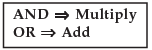2) The above fundamental principles may be generalised, wherever necessary.

Definition : The factorial n, written as n! or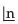, represents the product of all integers from 1 to n both inclusive. To make the notation meaningful, when n = o, we define o! or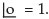Thus, n! = n (n – 1) (n – 2) ….. …3.2.1

Example 1 : Find 5! ; 4! and 6!

Solution : 5! = 5 × 4 × 3 × 2 × 1 = 120; 4! = 4 × 3 × 2 × 1 = 24; 6! = 6 × 5 × 4 × 3 × 2 × 1 = 720.

Example 2 : Find 9 ! / 6 ! ; 10 ! / 7 !.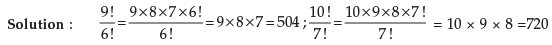Example 3 : Find x if 1/9 ! + 1/10 ! = x/11 !

Solution : 1/9! (1 + 1/10) = x/11 × 10 × 9! Or, 11/10 = x/11 × 10 i.e., x = 121

Example 4 : Find n ifSolution: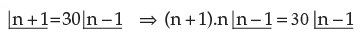or, n2 + n = 30 or, n2 + n – 30 or, n2 + 6n – 5n – 30 = 0      or,   (n + 6) (n – 5) = 0 either n = 5 or n = –6. (Not possible) ∴ n = 5.

5.3 PERMUTATIONS

A group of persons want themselves to be photographed. They approach the photographer and request him to take as many different photographs as possible with persons standing in different positions amongst themselves. The photographer wants to calculate how many films does he need to exhaust all possibilities? How can he calculate the number?
In the situations such as above, we can use permutations to find out the exact number of films.

Definition: The ways of arranging or selecting smaller or equal number of persons or objects from a group of persons or collection of objects with due regard being paid to the order of arrangement or selection, are called permutations.
Let us explain, how the idea of permutation will help the photographer. Suppose the group consists of Mr. Suresh, Mr. Ramesh and Mr. Mahesh. Then how many films does the photographer need? He has to arrange three persons amongst three places with due regard to order. Then the various possibilities are (Suresh, Mahesh, Ramesh), (Suresh, Ramesh, Mahesh), (Ramesh, Suresh, Mahesh), (Ramesh, Mahesh, Suresh), (Mahesh, Ramesh, Suresh) and (Mahesh, Suresh, Ramesh ). Thus there are six possibilities. Therefore he needs six films. Each one of these possibilities is called permutation of three persons taken at a time.

This may also be exhibited as follows :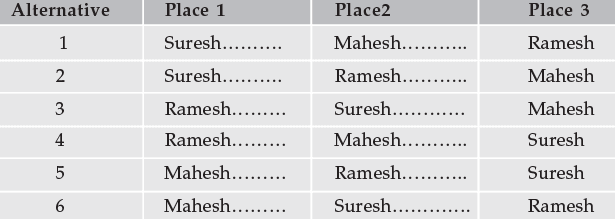with this example as a base, we can introduce a general formula to find the number of permutations.

Number of Permutations when r objects are chosen out of n different objects. (Denoted by nPr or nPr or P(n, r) ) :

Let us consider the problem of finding the number of ways in which the first r rankings are secured by n students in a class. As any one of the n students can secure the first rank, the number of ways in which the first rank is secured is n.

Now consider the second rank. There are (n – 1) students left, the second rank can be secured by any one of them. Thus the different possibilities are (n – 1) ways. Now, applying fundamental principle, we can see that the first two ranks can be secured in n (n – 1) ways by these n students.
After calculating for two ranks, we find that the third rank can be secured by any one of the remaining (n – 2) students. Thus, by applying the generalized fundamental principle, the first three ranks can be secured in n (n – 1) (n – 2) ways .

Continuing in this way we can visualise that the number of ways are reduced by one as the rank is increased by one. Therefore, again, by applying the generalised fundamental principle for r different rankings, we calculate the number of ways in which the first r ranks are secured by n students as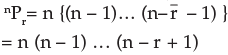Theorem: The number of permutations of n things chosen r at a time is given by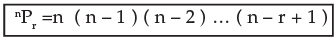where the product has exactly r factors.

5.4 RESULTS

1. Number of permutations of n different things taken all n things at a time is given by

nPn = n (n – 1) (n – 2) …. (n – n + 1)
=n (n – 1) (n – 2) ….. 2.1 = n!

2. nPr using factorial notation. nPr = n. (n – 1) (n – 2) ….. (n – r + 1)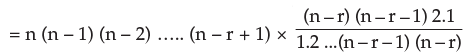= n!/( n – r )!
Thus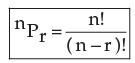3. Justification for 0! = 1. Now applying r = n in the formula for nPr, we get
nPn = n!/ (n – n)! = n!/0!
But from Result 1 we find that nPn = n!. Therefore, by applying this we derive, 0! = n! / n! = 1

Example 1 : Evaluate each of 5P3, 10P2, 11P5.

Solution : 5P3 = 5×4× (5–3+1) = 5 × 4 × 3 = 60,
10P2 = 10 × …. × (10–2+1) = 10 × 9 = 90,
11P= 11! / (11 – 5)! = 11 × 10 × 9 × 8 × 7 × 6! / 6! = 11 × 10 × 9 × 8 × 7 = 55440.

Example 2 : How many three letters words can be formed using the letters of the words (a) square and (b) hexagon?

(Any arrangement of letters is called a word even though it may or may not have any meaning or pronunciation).

Solution : (a) Since the word ‘square’ consists of 6 different letters, the number of permutations of choosing 3 letters out of six equals 6P3 = 6 × 5 × 4 = 120.

(b) Since the word ‘hexagon’ contains 7 different letters, the number of  permutations is 7P3 = 7 × 6 × 5 = 210.

Example 3 : In how many different ways can five persons stand in a line for a group photograph?

Solution : Here we know that the order is important. Hence, this is the number of permutations of five things taken all at a time. Therefore, this equals
5P5 = 5! = 5 × 4 × 3 × 2 × 1 = 120 ways.

Example 4 : First, second and third prizes are to be awarded at an engineering fair in which 13 exhibits have been entered. In how many different ways can the prizes be awarded?

Solution : Here again, order of selection is important and repetitions are not meaningful as no one can receive more than one prize. Hence , the answer is the number of permutations of 13 things chosen three at a time. Therefore, we find 13P= 13!/10! = 13×12×11 = 1,716 ways.

Example 5 : In how many different ways can 3 students be associated with 4 chartered accountants, assuming that each chartered accountant can take at most one student?

Solution : This equals the number of permutations of choosing 3 persons out of 4. Hence , the answer is 4P3 = 4×3×2 = 24.

Example 6 : If six times the number permutations of n things taken 3 at a time is equal to seven times the number of permutations of (n – 1) things chosen 3 at a time, find n.

Solution : We are given that 6 × nP3 = 7 × n-1P3 and we have to solve this equality to find the value of n. Therefore,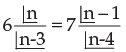or, 6 n (n – 1) (n – 2) = 7 (n – 1) (n – 2) (n – 3)
or, 6 n = 7 (n – 3)
or, 6 n = 7n – 21
or, n = 2 1
Therefore, the value of n equals 21.

Example 7 : Compute the sum of 4 digit numbers which can be formed with the four digits 1, 3, 5, 7, if each digit is used only once in each arrangement.
Solution : The number of arrangements of 4 different digits taken 4 at a time is given by 4P4 = 4! = 24. All the four digits will occur equal number of times at each of the position, namely ones, tens, hundreds, thousands.
Thus, each digit will occur 24 / 4 = 6 times in each of the position. The sum of digits in one’s position will be 6 × (1 + 3 + 5 + 7) = 96. Similar is the case in ten’s, hundred’s and thousand’s places. Therefore, the sum will be 96 + 96 × 10 + 96 × 100 + 96 × 1000 = 106656.

Example 8 : Find n if nP3 = 60.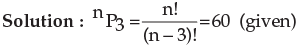i.e., n (n–1) (n–2) = 60 = 5 × 4 × 3
Therefore, n = 5.

Example 9 : If 56Pr+6 : 54Pr+3 = 30800 : 1, find r.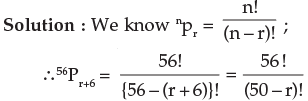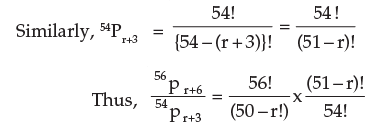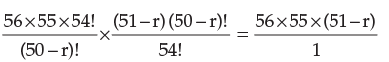But we are given the ratio as 30800 : 1 ; therefore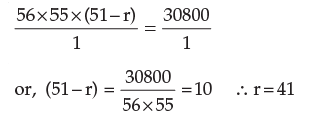Example 10 : Prove the following
(n + 1)! – n! = ⇒ n.n!

Solution : By applying the simple properties of factorial, we have
(n +1)! – n! = (n+1) n! – n! = n!. (n+1–1) = n. n!

Example 11 : In how many different ways can a club with 10 members select a President, Secretary and Treasurer, if no member can hold two offices and each member is eligible for any office?

Solution : The answer is the number of permutations of 10 persons chosen three at a time.
Therefore, it is 10p3 = 10×9×8=720.

Example 12 : When Jhon arrives in New York, he has eight shops to see, but he has time only to visit six of them. In how many different ways can he arrange his schedule in New York?

Solution : He can arrange his schedule in 8P6 = 8× 7 × 6 × 5 × 4 × 3 = 20160 ways.

Example 13 : When Dr. Ram arrives in his dispensary, he finds 12 patients waiting to see him.
If he can see only one patients at a time, find the number of ways, he can schedule his patients (a) if they all want their turn, and (b) if 3 leave in disgust before Dr. Ram gets around to seeing them.

Solution : (a) There are 12 patients and all 12 wait to see the doctor. Therefore the number of ways = 12P12 = 12! = 479,001,600

(b) There are 12–3 = 9 patients. They can be seen 12P9 = 79,833,600 ways.

5.5 CIRCULAR PERMUTATIONS

So for we have discussed arrangements of objects or things in a row which may be termed as linear permutation. But if we arrange the objects along a closed curve viz., a circle, the permutations are known as circular permutations.

The number of circular permutations of n different things chosen at a time is (n–1)!.
Proof : Let any one of the permutations of n different things taken. Then consider the rearrangement of this permutation by putting the last thing as the first thing. Eventhough this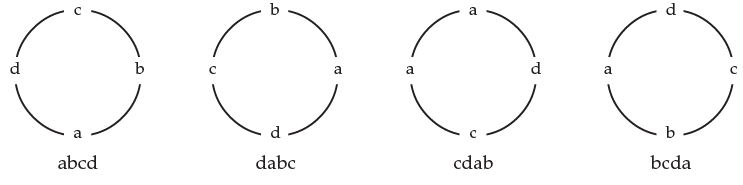is a different permutation in the ordinary sense, it will not be different in all n things are arranged in a circle. Similarly, we can consider shifting the last two things to the front and so on. Specially, it can be better understood, if we consider a,b,c,d. If we place a,b,c,d in order, then we also get abcd, dabc, cdab, bcda as four ordinary permutations. These four words in circular case are one and same thing. See above circles.

Thus we find in above illustration that four ordinary permutations equals  one in circular.
Therefore, n ordinary permutations equal one circular permutation.
Hence there are nPn/ n ways in which all the n things can be arranged in a circle. This equals (n–1)!.

Example 1 : In how many ways can 4 persons sit at a round table for a group discussions?

Solution : The answer can be get from the formula for circular permutations. The answer is (4–1)! = 3! = 6 ways.

NOTE :  These arrangements are such that every person has got the same two neighbours. The only change is that right side neighbour and vice-versa.
Thus the number of ways of arranging n persons along a round table so that no person has the same two neighbours is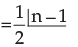Similarly, in forming a necklace or a garland there is no distinction between a clockwise and anti clockwise direction because we can simply turn it over so that clockwise becomes anti clockwise and vice versa. Hence, the number of necklaces formed with n beads of different colours5.6 PERMUTATION WITH RESTRICTIONS

In many arrangements there may be number of restrictions. in such cases, we are to arrange or select the objects or persons as per the restrictions imposed. The total number of arrangements in all cases, can be found out by the application of fundamental principle.

Theorem 1. Number of permutations of n distinct objects when a particular object is not taken in any arrangement is n–1pr.

Proof : Since a particular object is always to be excluded, we have to place n – 1 objects at r places. Clearly this can be done in n–1pr ways.

Theorem 2. Number of permutations of n distinct objects when a particular object is always included in any arrangement is r. n–1pr–1.

Proof : If the particular object is placed at first place, remaining r – 1 places can be filled from n – 1 distinct objects in n–1pr–1 ways. Similarly, by placing the particular object in 2nd, 3rd, ….., rth place, we find that in each case the number of permutations is n–1pr–1.This the total number of arrangements in which a particular object always occurs is r. n–1pr–1 The following examples will enlighten further:

Example 1 : How many arrangements can be made out of the letters of the word DRAUGHT, the vowels never beings separated?

Solution : The word DRAUGHT consists of 7 letters of which 5 are consonants and two are vowels. In the arrangement we are to take all the 7 letters but the restriction is that the two vowels should not be separated.

We can view the two vowels as one letter. The two vowels A and U in this one letter can be arranged in 2! = 2 ways. (i) AU or (ii) UA. Further, we can arrange the six letters : 5 consonants and one letter compound letter consisting of two vowels. The total number of ways of arranging them is 6P = 6! = 720 ways.
Hence, by the fundamental principle, the total number of arrangements of the letters of the word DRAUGHT, the vowels never being separated = 2 × 720 = 1440 ways.

Example 2 : Show that the number of ways in which n books can be arranged on a shelf so that two particular books are not together.The number is (n–2).(n–1)!

Solution: We first find the total number of arrangements in which all n books can be arranged on the shelf without any restriction. The number is,nPn = n! ….. (1)
Then we find the total number of arrangements in which the two particular books are together.

The books can be together in 2P2 = 2! = 2 ways. Now we consider those two books which are kept together as one composite book and with the rest of the (n–2) books from (n–1) books which are to be arranged on the shelf; the number of arrangements = n–1Pn–1 = (n–1) !. Hence by the Fundamental Principle, the total number of arrangements on which the two particular books are together equals = 2 × (n–1)! …….(2)
the required number of arrangements of n books on a shelf so that two particular books are not together
= (1) – (2)
= n! – 2 x (n–1)!
= n.(n – 1)! – 2 . (n–1)!
=(n–1)! . (n–2)

Example 3 : There are 6 books on Economics, 3 on Mathematics and 2 on Accountancy. In how many ways can these be placed on a shelf if the books on the same subject are to be together?

Solution : Consider one such arrangement. 6 Economics books can be arranged among themselves in 6! Ways, 3 Mathematics books can be arranged in 3! Ways and the 2 books on Accountancy can be arranged in 2! ways. Consider the books on each subject as one unit. Now there are three units. These 3 units can be arranged in 3! Ways.
Total number of arrangements =  3! × 6! × 3! × 2!
=  51,840.

Example 4 : How many different numbers can be formed by using any three out of five digits 1, 2, 3, 4, 5, no digit being repeated in any number?
How many of these will (i) begin with a specified digit? (ii) begin with a specified digit and end with another specified digit?

Solution : Here we have 5 different digits and we have to find out the number of permutations of them 3 at a time. Required number is 5P3 = 5.4.3 = 60.
(i) If the numbers begin with a specified digit, then we have to find

The number of Permutations of the remaining 4 digits taken 2 at a time. Thus, desire number is 4P2 = 4.3 = 12.
(ii) Here two digits are fixed; first and last; hence, we are left with the choice of finding the number of permutations of 3 things taken one at a time i.e., 3P1 =3.

Example 5 : How many four digit numbers can be formed out of the digits 1,2,3,5,7,8,9, if no digit is repeated in any number? How many of these will be greater than 3000?

Solution : We are given 7 different digits and a four-digit number is to be formed using any 4 of these digits. This is same as the permutations of 7 different things taken 4 at a time.
Hence, the number of four-digit numbers that can be formed = 7P4 = 7 × 6 × 5 × 4 × = 840 ways.
Next, there is the restriction that the four-digit numbers so formed must be greater than 3,000. thus, it will be so if the first digit-that in the thousand’s position, is one of the five digits 3, 5, 7, 8, 9. Hence, the first digit can be chosen in 5 different ways; when this is done, the rest of the 3 digits are to be chosen from the rest of the 6 digits without any restriction and this can be done in 6P3 ways.
Hence, by the Fundamental principle, we have the number of four-digit numbers greater than 3,000 that can be formed by taking 4 digits from the given 7 digits = 5 × 6P= 5 × 6 × 5 × 4 = 5 × 120 = 600.

Example 6 : Find the total number of numbers greater than 2000 that can be formed with the digits 1, 2, 3, 4, 5 no digit being repeated in any number.

Solution : All the 5 digit numbers that can be formed with the given 5 digits are greater than 2000. This can be done in
5P5 = 5! = 120 ways …...................................(1)
The four digited numbers that can be formed with any four of the given 5 digits are greater than 2000 if the first digit, i.e.,the digit in the thousand’s position is one of the four digits 2, 3, 4, 5. this can be done in 4P1 = 4 ways. When this is done, the rest of the 3 digits are to be chosen from the rest of  5–1 = 4 digits. This can be done in 4P3 = 4 × 3 × 2 = 24 ways.
Therefore, by the Fundamental principle, the number of four-digit numbers greater than 2000 = 4 × 24 = 96 …. (2)
Adding (1) and (2), we find the total number greater than 2000 to be 120 + 96 = 216.

Example 7 : There are 6 students of whom 2 are Indians, 2 Americans, and the remaining 2 are Russians. They have to stand in a row for a photograph so that the two Indians are together, the two Americans are together and so also the two Russians. Find the number of ways in which they can do so.

Solution : The two Indians can stand together in 2P2 = 2! = 2 ways. So is the case with the two Americans and the two Russians.
Now these 3 groups of 2 each can stand in a row in 3P3 = 3 x 2 = 6 ways. Hence by the generalized fundamental principle, the total number of ways in which they can stand for a photograph under given conditions is 6 × 2 × 2 × 2 = 48

Example 8 : A family of 4 brothers and three sisters is to be arranged for a photograph in one row. In how many ways can they be seated if (i) all the sisters sit together, (ii) no two sisters sit together?

Solution : (i) Consider the sisters as one unit and each brother as one unit. 4 brother and 3 sisters make 5 units which can be arranged in 5! ways. Again 3 sisters may be arranged amongst themselves in 3! Ways Therefore, total number of ways in which all the sisters sit together = 5!×3! = 720 ways.
(ii) In this case, each sister must sit on each side of the brothers. There are 5 such positions as indicated below by upward arrows :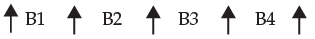4 brothers may be arranged among themselves in 4! ways. For each of these arrangements 3 sisters can sit in the 5 places in 5P3 ways.
Thus the total number of ways = 5P3 × 4! = 60 × 24 = 1,440

Example 9 : In how many ways can 8 persons be seated at a round table? In how many cases will 2 particular persons sit together?

Solution : This is in form of circular permutation. Hence the number of ways in which eight persons can be seated at a round table is (n – 1)! = (8 – 1)! = 7! = 5040 ways.
Consider the two particular persons as one person. Then the group of 8 persons becomes a group of 7 (with the restriction that the two particular persons be together) and seven persons can be arranged in a circular in 6! Ways.
Hence, by the fundamental principle, we have, the total number of cases in which 2 particular persons sit together in a circular arrangement of 8 persons = 2! × 6! = 2 × 6 × 5 × 4 × 3 ×2×1 = 1,440.

Example 10 : Six boys and five girls are to be seated for a photograph in a row such that no two girls sit together and no two boys sit together. Find the number of ways in which this can be done.

Solution : Suppose that we have 11 chairs in a row and we want the 6 boys and 5 girls to be seated such that no two girls and no two boys are together. If we number the chairs from left to right, the arrangement will be possible if and only if boys occupy the odd places and girls occupy the even places in the row. The six odd places from 1 to 11 may filled in by 6 boys in 6P6 ways. Similarly, the five even places from 2 to 10 may be filled in by 5 girls in 5P5 ways.
Hence, by the fundamental principle, the total number of required arrangements = 6P6 × 5P5 = 6! × 5! = 720 × 120 = 86400.

5.7 COMBINATIONS

We have studied about permutations in the earlier section. There we have said that while arranging or selecting, we should pay due regard to order. There are situations in which order is not important. For example, consider selection of 5 clerks from 20 applicants. We will not be concerned about the order in which they are selected. In this situation, how to find the number of ways of selection? The idea of combination applies here.

Definition : The number of ways in which smaller or equal number of things are arranged or selected from a collection of things where the order of selection or arrangement is not important, are called combinations.

The selection of a Poker hand which is a combination of five cards selected from 52 cards is an example of combination of 5 things out of 52 things.

Number of combinations of n different things taken r at a time. (denoted by nCr  C(n,r)  C (n/r ), Cn,r)

Let nCr denote the required number of combinations. Consider any one of those combinations.
It will contain r things. Here we are not paying attention to order of selection. Had we paid attention to this, we will have permutations or r items taken r at a time. In other words, every combination of r things will have rPr permutations amongst them. Therefore, nCr combinations will give rise to nCr. rPr permutations of r things selected form n things. From the earlier section, we can say that nCr. rPr = nPr as nPr denotes the number of permutations of r things chosen out of n things.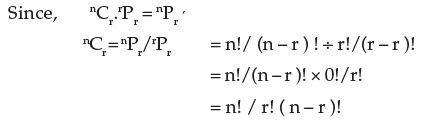Remarks: Using the above formula, we get
(i) nCo = n! / 0! ( n – 0 )! = n!/n! =1. [ As 0! = 1]
nC= n! / n! ( n – n ) ! = n! / n! 0! = 1 [ Applying the formula for nCr  with r = n ]

Example 1 : Find the number of different poker hands in a pack of 52 playing cards.

Solution : This is the number of combinations of 52 cards taken five at a time. Now applying the formula,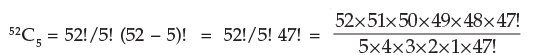= 2,598,960

Example 2 : Let S be the collection of eight points in the plane with no three points on the straight line. Find the number of triangles that have points of S as vertices.

Solution : Every choice of three points out of S determine a unique triangle. The order of the points selected is unimportant as whatever be the order, we will get the same triangle. Hence, the desired number is the number of combinations of eight things taken three at a time.
Therefore, we get
8C3 = 8!/3!5! = 8×7×6/3×2×1 = 56 choices.

Example 3 : A committee is to be formed of 3 persons out of 12. Find the number of ways of forming such a committee.

Solution : We want to find out the number of combinations of 12 things taken 3 at a time and this is given by

12C3 = 12!/3!(12 – 3)! [ by the definition of nCr]
= 12!/3!9! = 12×11×10×9!/3!9! = 12×11×10/3×2 = 220

Example 4 : A committee of 7 members is to be chosen from 6 Chartered Accountants, 4 Economists and 5 Cost Accountants. In how may ways can this be done if in the committee, there must be at least one member from each group and at least 3 Chartered Accountants?

Solution : The various methods of selecting the persons from the various groups are shown below: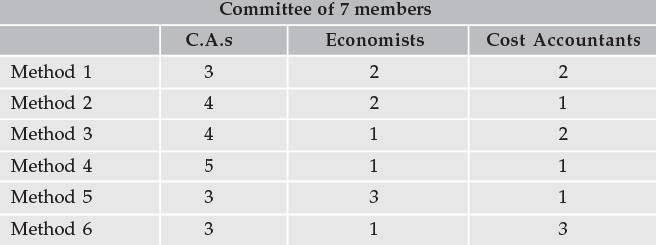Number of ways of choosing the committee members by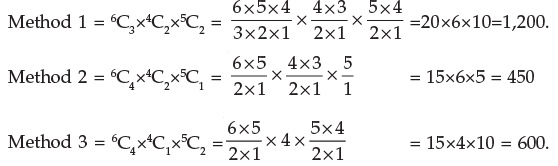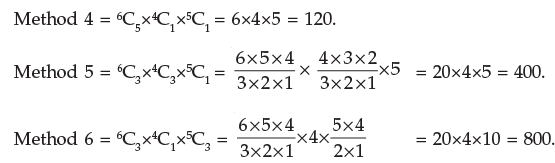Therefore, total number of ways = 1,200 + 450 + 600 + 120 + 400 + 800 = 3,570

Example 5: A person has 12 friends of whom 8 are relatives. In how many ways can he invite 7 guests such that 5 of them are relatives?

Solution : Of the 12 friends, 8 are relatives and the remaining 4 are not relatives. He has to invite 5 relatives and 2 friends as his guests. 5 relatives can be chosen out of 8 in 8C5 ways; 2 friends can be chosen out of 4 in 4C2 ways.

Hence, by the fundamental principle, the number of ways in which he can invite 7 guests such that 5 of them are relatives and 2 are friends.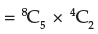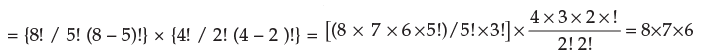= 336.

Example 6 : A Company wishes to simultaneously promote two of its 6 department heads out of 6 to assistant managers. In how many ways these promotions can take place?

Solution : This is a problem of combination. Hence, the promotions can be done in
6C2 = 6×5 / 2 = 15 ways

Example 7 : A building contractor needs three helpers and ten men apply. In how many ways can these selections take place?

Solution : There is no regard for order in this problem. Hence, the contractor can select in any of 10C3 ways i.e.,
(10 × 9 × 8) / (3 × 2 × 1) = 120 ways.

Example 8: In each case, find n:

Solution : (a) 4. nC2 = n+2 C3; (b) n+2 Cn = 45.
(a) We are given that 4. nC2 = n+2 C3. Now applying the formula,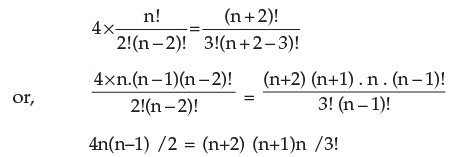or, 4n(n–1) / 2 = (n+2)(n+1)n /3×2×1
or, 12(n–1)=(n+2) (n+1)
or, 12n–12 = n2 + 3n +2
or, n2 – 9n + 14 = 0.
or, n2 – 2n – 7n + 14 = 0.
or, (n–2) (n–7) = 0
∴ n=2   or   7.

(b) We are given that n+2Cn = 45. Applying the formula,
(n+2)!/{n!(n+2–n)!} = 45
or, (n+2) (n+1) n! / n! 2! = 45

or, (n+1) (n+2) = 45×2! = 90
or, n2+3n–88 = 0
or, n2+11n–8n–88 = 0
or, (n+11) (n–8) = 0

Thus, n equals either – 11 or 8. But negative value is not possible. Therefore we conclude that n=8.

Example 9 : A box contains 7 red, 6 white and 4 blue balls. How many selections of three balls can be made so that (a) all three are red, (b) none is red, (c) one is of each colour?

Solution : (a) All three balls will be of red colour if they are taken out of 7 red balls and this can be done in
7C3 = 7! / 3!(7–3)!
= 7! / 3!4! = 7×6×5×4! / (3×2×4!) = 7×6×5 / (3×2) = 35 ways
Hence, 35 selections (groups) will be there such that all three balls are red.
(b) None of the three will be red if these are chosen from (6 white and 4 blue balls) 10 balls and this can be done in
10C3 =  10!/{3!(10–3)!} = 10! / 3!7!
=  10×9×8×7! / (3×2×1×7!) = 10×9×8 /(3×2) = 120 ways.
Hence, the selections (or groups) of three such that none is red ball are 120 in number.
One red ball can be chosen from 7 balls in 7C1 = 7 ways. One white ball can be chosen from 6 white balls in 6C1 ways. One blue ball can be chosen from 4 blue balls in 4C= 4 ways. Hence, by generalized fundamental principle, the number of groups of three balls such that one is of each colour = 7×6×4 = 168 ways.

Example 10 : If 10Pr = 604800 and 10Cr = 120; find the value of r,

Solution : We know that nCr. rPr = nPr. We will us this equality to find r.
10Pr = 10C.r!
or, 604800 = 120 ×r!
or, r! = 604800 ÷ 120 = 5040
But r! = 5040 = 7×6×4×3×2×1 = 7!
Therefore, r=7.

Properties of nCr :
1. nCr = nCn–r
We have nC= n! / {r!(n–r)!} and nCn–r = n! / [(n–r)! {n–(n–r)}!] = n! / {(n–r)!(n–n+r)!}
Thus nCn–r = n! / {(n–r)! (n–n+r)!} = n! / {(n–r)!r!} = nCr

2. n+1C= nC+ nCr–1

By definition,
nCr–1 + nCr = n! / {(r–1)! (n–r+1)!} + n! / {r!(n–r)!}
But r! = r×(r–1)! and (n–r+1)! = (n–r+1) × (n–r)!. Substituting these in above, we get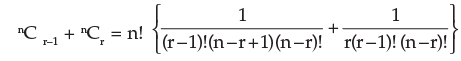= {n! / (r–1)! (n–r)!} {(1 / n–r+1) + (1/r) }
= {n! / (r–1)! (n–r)!} {(r+n–r+1) / r(n–r+1) }
= (n+1) n! / {r . (r–1)! (n–r)! . (n–r+1)}
= (n+1)! / {r!(n+1–r)!} = n+1Cr

3. nCo = n!/{0! (n–0)!} = n! / n! =1.
4. nCn = n!/{n! (n–n)!} = n! / n! . 0! = 1.

Note
(a) nCr has a meaning only when 0≤ r ≤ n, nCn–r has a meaning only when 0 ≤ n – r ≤ n.
(b) nCr and nCn–r are called complementary combinations, for if we form a group of r things out of n different things, (n–r) remaining things which are not included in this group form another group of rejected things. The number of groups of n different things, taken r at a time should be equal to the number of groups of n different things taken (n–r) at a time.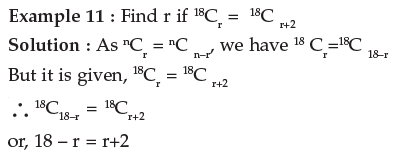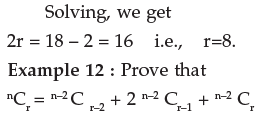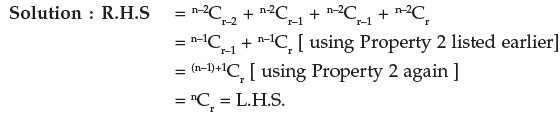Hence, the result

Example 13 : If 28C2r : 24C2r–4 = 225 : 11, find r.

Solution : We have nCr = n! / {r!(n–r)!} Now, substituting for n and r, we get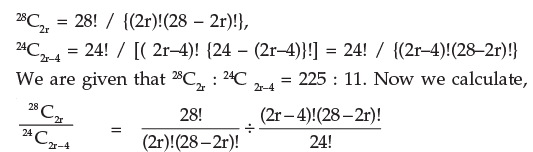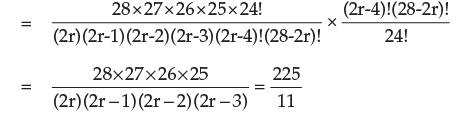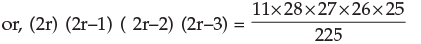= 11×28×3×26
= 11×7×4×3×13×2
= 11×12×13×14
= 14×13×12×11
∴   2r= 14 i.e., r = 7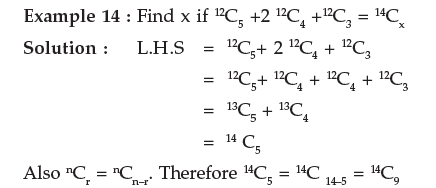Hence, L.H.S = 14C5 = 14C= 14Cx = R.H.S by the given equality
This implies, either x = 5 or x = 9.

Example 15 : Prove by reasoning that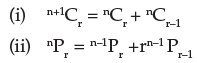Solution : (i) n+1Cr stands for the number of combinations of (n+1) things taken r at a time. As a specified thing can either be included in any combination or excluded from it, the total number of combinations which can be combinations or (n+1) things taken r at a time is the sum of :

(a) combinations of (n+1) things taken r at time in which one specified thing is always included and
(b) the number of combinations of (n+1) things taken r at time from which the specified thing is always excluded.
Now, in case (a), when a specified thing is always included , we have to find the number of ways of selecting the remaining (r–1) things out of the remaining n things which is nCr–1.
Again, in case (b), since that specified thing is always excluded, we have to find the number of ways of selecting r things out of the remaining n things,  which is nCr.
Thus, n+1Cr = nCr–1+ nCr
(i) We devide nPi.e., the number of permutations of n things take r at a time into two groups:
(a) those which contain a specified thing
(b) those which do not contain a specified thing.
In (a) we fix the particular thing in any one of the r places which can be done in r ways and then fill up the remaining (r–1) places out of (n–1) things which give rise to n–1 Pr–1 ways. Thus, the number of permutations in case (a) = r × n–1Pr–1.
In case (b), one thing is to be excluded; therefore, r places are to be filled out of (n–1) things.
Therefore, number of permutations = n–1Pr
Thus, total number of permutations = n–1Pr + r. n–1Pr–1
i.e.,   nPr = n–1Pr+r. n–1Pr–1

5.8 STANDARD RESULTS

We now proceed to examine some standard results in permutations and combinations. These results have special application and hence are dealt with separately.

I. Permutations when some of the things are alike, taken all at a time
The number of ways p in which n things may be arranged among themselves, taking them all at a time, when n1 of the things are exactly alike of one kind , nof the things are exactly alike of another kind, n3 of the things are exactly alike of the third kind, and the rest all are different is given by,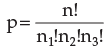Proof : Let there be n things. Suppose n1 of them are exactly alike of one kind; n2 of them are exactly alike of another kind; n3 of them are exactly alike of a third kind; let the rest (n–n1–n2–n3) be all different.
Let p be the required permutations; then if the n things, all exactly alike of one kind were replaced by n, different things different from any of the rest in any of the p permutations without altering the position of any of the remaining things, we could form n1! new permutations.
Hence, we should obtain p × n1! permutations.
Similarly if n2 things exactly alike of another kind were replaced by n2 different things different form any of the rest, the number of permutations would be p × n1! × n2!

Similarly, if n3 things exactly alike of a third kind were replaced by n3 different things different from any of the rest, the number of permutations would be p × n1! × n2! × n3! = n!
But now because of these changes all the n things are different and therefore, the possible number of permutations when all of them are taken is n!.
Hence,   p×n1! × n2! n3! = n!

i.e.,which is the required number of permutations. This results may be extended to cases where there are different number of groups of alike things.

II. Permutations when each thing may be repeated once, twice,…upto r times in any arrangement.

Result: The number of permutations of n things taken r at time when each thing may be repeated r times in any arrangement is nr.

Proof: There are n different things and any of these may be chosen as the first thing. Hence, there are n ways of choosing the first thing.
When this is done, we are again left with n different things and any of these may be chosen as the second (as the same thing can be chosen again.) Hence, by the fundamental principle, the two things can be chosen in n × n = nnumber of ways.
Proceeding in this manner, and noting that at each stage we are to chose a thing from n different things, the total number of ways in which r things can be chosen is obviously equal to n × n × ………to r terms = nr.

III. Combinations of n different things taking some or all of n things at a time

Result : The total number of ways in which it is possible to form groups by taking some or all of n things (2n –1).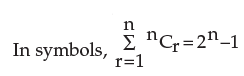Proof : Each of the n different things may be dealt with in two ways; it may either be taken or left. Hence, by the generalised fundamental principle, the total number of ways of dealing with n things :
2 × 2 × 2×……..2, n times i.e., 2n
But this include the case in which all the things are left, and therefore, rejecting this case, the total number of ways of forming a group by taking some or all of n different things is 2n – 1.
IV. Combinations of n things taken some or all at a time when n1 of the things are alike of one kind, n2 of the things are alike of another kind n3 of the things are alike of a third kind. etc.

Result : The total, number of ways in which it is possible to make groups by taking some or all out of n (=n1 + n2 + n3 +…) things, where n1 things are alike of one kind and so on, is given by {(n1 + 1) ( n+ 1) ( n+ 1)…} –1
Proof : The n1 things all alike of one kind may be dealt with in (n+ 1) ways. We may take 0, 1, 2,….n, of them. Similarly n2 things all alike of a second kind may be dealt with in (n2 +1) ways and n3 things all alike of a third kind may de dealt with in (n3 +1) ways.
Proceeding in this way and using the generalised fundamental principle, the total number of ways of dealing with all n ( = n1 + n2 + n3 +…) things, where n1, things are alike of one kind and so on, is given by (n1 + 1) ( n2 + 1) ( n+ 1)…
But this includes the case in which none of the things are taken. Hence, rejecting this case, total number of ways is {(n1 + 1) ( n2 + 1) ( n3 + 1)…} –1}

V. The notion of Independence in Combinations

Many applications of Combinations involve the selection of subsets from two or more independent sets of objects or things. If the combination of a subset having r1 objects form a set having n1 objects does not affect the combination of a subset having r2 objects from a different set having nobjects, we call the combinations as being independent. Whenever such combinations are independent, any subset of the first set of objects can be combined with each subset of the second set of the object to form a bigger combination. The total number of such combinations can be found by applying the generalised fundamental principle.
Result : The combinations of selecting r1 things from a set having nobjects and rthings from a set having n2 objects where combination of rthings, r2 things are independent is given by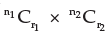Note : This result can be extended to more than two sets of objects by a similar reasoning.

Example 1 : How many different permutations are possible from the letters of the word CALCULUS?

Solution: The word CALCULUS consists of 8 letters of which 2 are C and 2 are L, 2 are U and the rest are A and S. Hence , by result (I), the number of different permutations from the letters of the word CALCULUS taken all at a time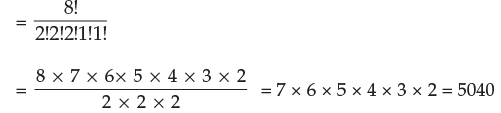Example 2 : In how many ways can 17 billiard balls be arranged , if 7 of them are black, 6 red and 4 white?

Solution : We have, the required number of different arrangements: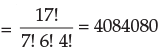Example 3 : An examination paper with 10 questions consists of 6 questions in Algebra and 4 questions in Geometry. At least one question from each section is to be attempted. In how many ways can this be done?

Solution : A student must answer atleast one question from each section and he may answer all questions from each section.
Consider Section I : Algebra. There are 6 questions and he may answer a question or may not answer it. These are the two alternatives associated with each of the six questions. Hence, by the generalised fundaments principle, he can deal with two questions in 2 × 2 ….6 factors = 26 number of ways. But this includes the possibility of none of the question from Algebra being attempted. This cannot be so, as he must attempt at least one question from this section. Hence, excluding this case, the number of ways in which Section I can be dealt with is (26 –1).
Similarly, the number of ways in which Section II can be dealt with is (24 –1).

Hence, by the Fundamental Principle, the examination paper can be attempted in (26 –1) (24 –1) number of ways.

Example 4 : A man has 5 friends. In how many ways can he invite one or more of his friends to dinner?

Solution : By result, (III) of this section, as he has to select one or more of his 5 friends, he can do so in 25 – 1 = 31 ways.
Note : This can also be done in the way, outlines below. He can invite his friends one by one, in twos, in threes, etc. and hence the number of ways.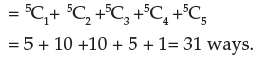Example 5 : There are 7 men and 3 ladies. Find the number of ways in which a committee of 6 can be formed of them if the committee is to include atleast two ladies?

Solution : The committee of six must include at least 2 ladies, i.e., two or more ladies. As there are only 3 ladies, the following possibilities arise: The committee of 6 consists of (i) 4 men and 2 ladies (ii) 3 men and 3 ladies.
The number of ways for (i) = 7C4 × 3C2 = 35 × 3 = 105;
The number of ways for (ii) = 7C3 × 3C3 = 35 × 1 = 35.
Hence the total number of ways of forming a committee so as to include at least two ladies = 105 +35 = 140.

Example 6 : Find the number of ways of selecting 4 letters from the word EXAMINATION.

Solution : There are 11 letters in the word of which A, I, N are repeated twice.

Thus we have 11 letters of 8 different kinds (A, A), (I, I), (N, N), E, X, M, T, O.
The group of four selected letters may take any of the following forms:
(i) Two alike and other two alike
(ii) Two alike and other two different
(iii) All four different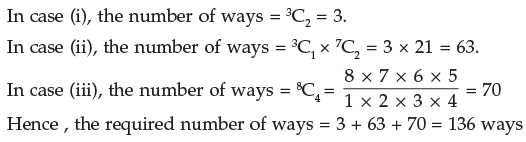The document Basic Concepts of Permutations and Combinations CA Foundation Notes | EduRev is a part of the CA Foundation Course Business Mathematics and Logical Reasoning & Statistics.
All you need of CA Foundation at this link: CA FoundationUse Code STAYHOME200 and get INR 200 additional OFF Use Coupon Code
All Tests, Videos & Notes of CA Foundation: CA Foundation## Business Mathematics and Logical Reasoning & Statistics

33 videos|101 docs|87 tests

Track your progress, build streaks, highlight & save important lessons and more!

,

,

,

,

,

,

,

,

,

,

,

,

,

,

,

,

,

,

,

,

,

;